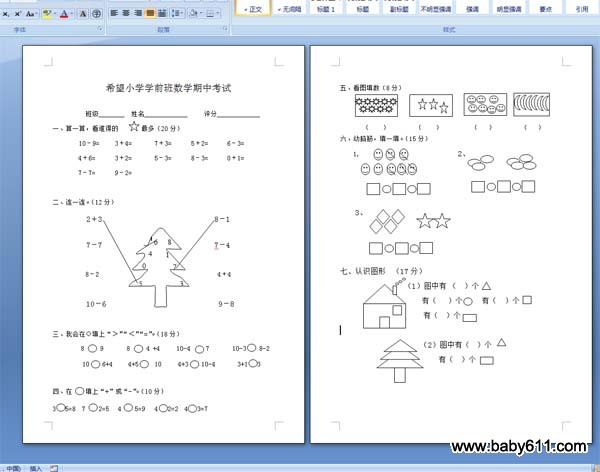# 希望小学学前班数学期中考试希望小学学前班数学期中考试班级          姓名                  评分一、算一算，看谁得的最多（20分）10－9=     3＋4=       7＋3=      5＋2=      6－3=4＋6=      3＋2=       5－3=      8－3=      0＋1=7－7=      9－2=二、连一连。（12分）2＋3                              8－17－7                              7－48－2                                    4＋410－6                              9－8三、我会在○填上"＞""＜""="。（18分）8     9       8     4 +4     10-4     7       10-3    8-210    6+4     4+5     10     4+3    10-4       3+1   3四、在    填上"+"或"-"。（10分）3   5=8  7    2=5   4    5=9   4   2=2  4   3=7五、看图填数（8分）（    ）        （    ）         （   ）        （   ）六、动脑筋，填一填。（15分）1.                             2、3、七、认识图形  （17分）（1）图中有（  ）个有（   ）个     有（  ）个有（  ）个（2）图中有（  ）个有（  ）个

此试卷格式为：WORD DOC格式，纸张：A4，页数：2页。

• 幼儿园试卷分类
• 将此试卷分享到：
• 数学最新更新